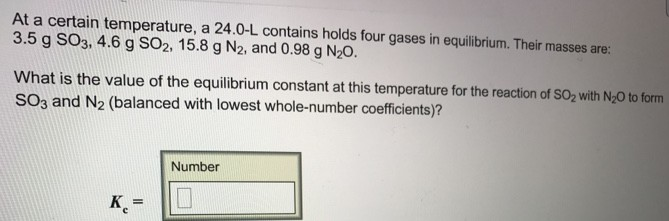# Problem: At a certain temperature a 24.0-L contains holds four gases in equilibrium. Their masses are: 3.5 g SO3, 4.6 g SO2, 15.8 g N2, and 0.98 g N2O. What is the value of the equilibrium constant at this temperature for the reaction of SO2 with N2O to form SO3 and N2 (balanced with lowest whole-number coefficients)?

###### FREE Expert Solution
80% (465 ratings)###### Problem Details

At a certain temperature a 24.0-L contains holds four gases in equilibrium. Their masses are: 3.5 g SO3, 4.6 g SO2, 15.8 g N2, and 0.98 g N2O.

What is the value of the equilibrium constant at this temperature for the reaction of SO2 with N2O to form SO3 and N2 (balanced with lowest whole-number coefficients)?